# RD Sharma Solutions for Class 8 Maths Chapter 21 Mensuration - II (Volumes and Surface Areas of a Cuboid and a Cube) Exercise 21.3

Exercise 21.3, mainly deals with the surface area of a cuboid and a cube. We shall also discuss the surface area of the walls of a room. Solutions here are formulated by our expert faculty team with utmost care. Students who are willing to clear their doubts and improve their problem-solving skills can make use of RD Sharma Class 8 Solutions. This is the best resource students can access and prepare for their exams. Students can download the PDF easily that is provided below.

## Download the Pdf of RD Sharma Solutions for Class 8 Maths Exercise 21.3 Chapter 21 Mensuration – II (Volumes and Surface Areas of a Cuboid and a Cube)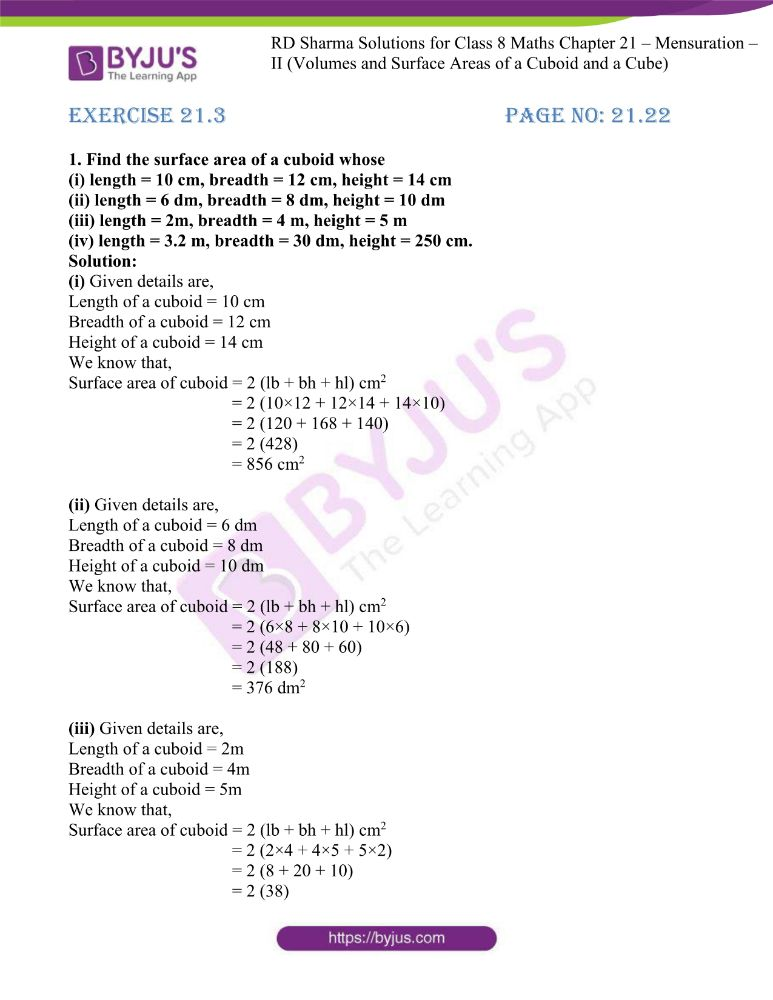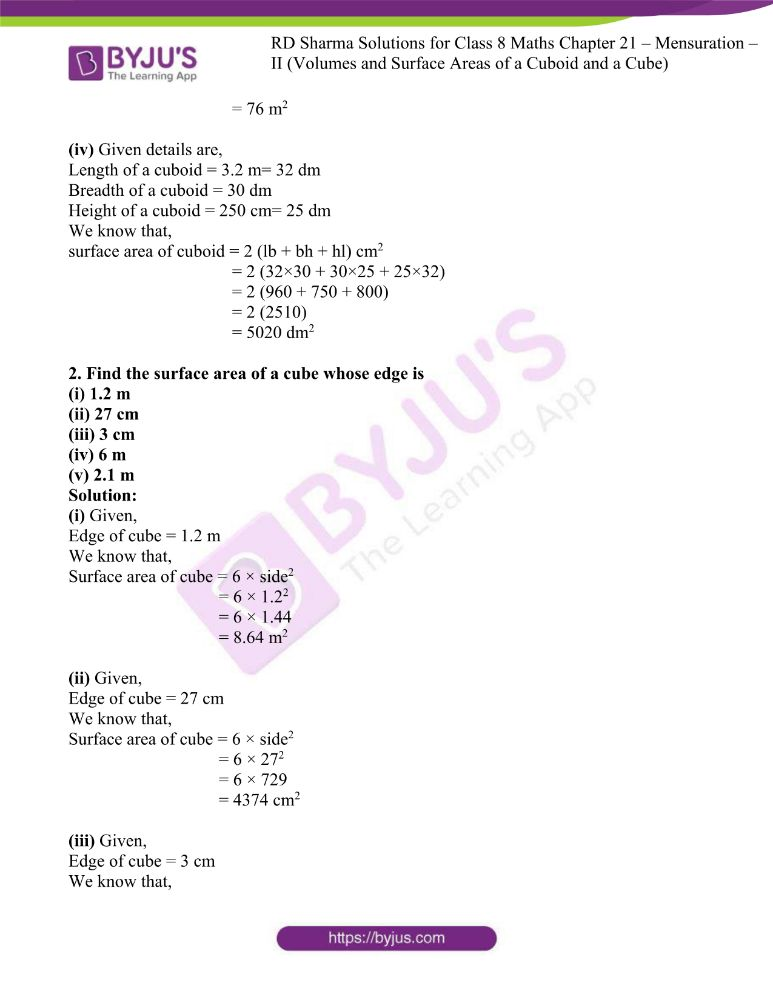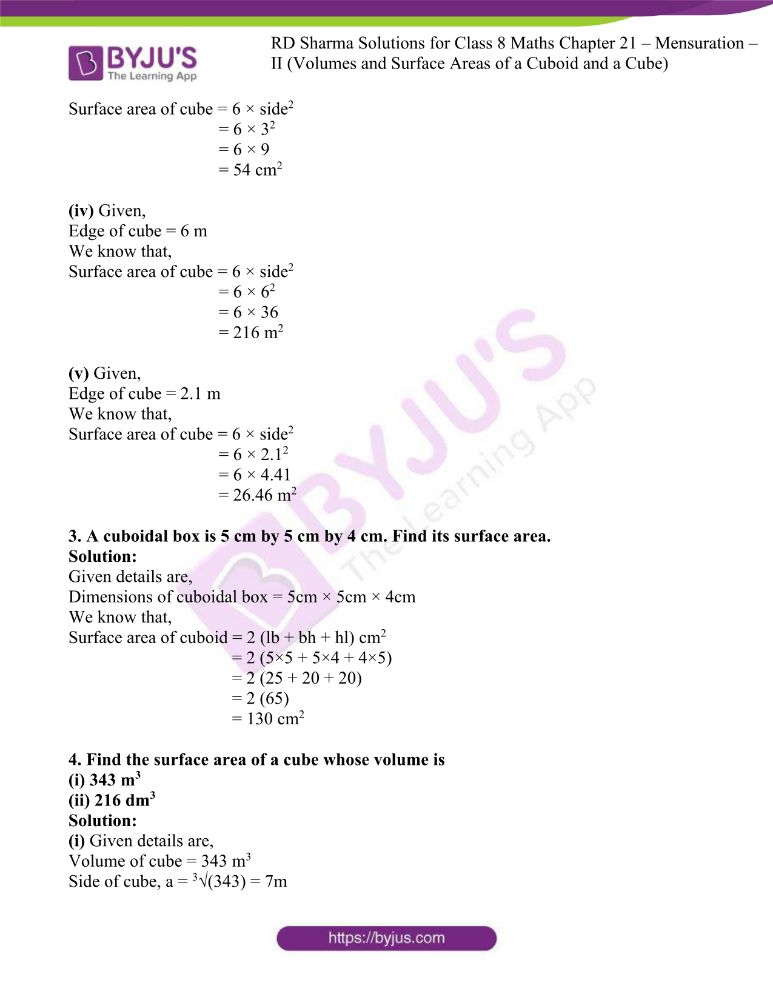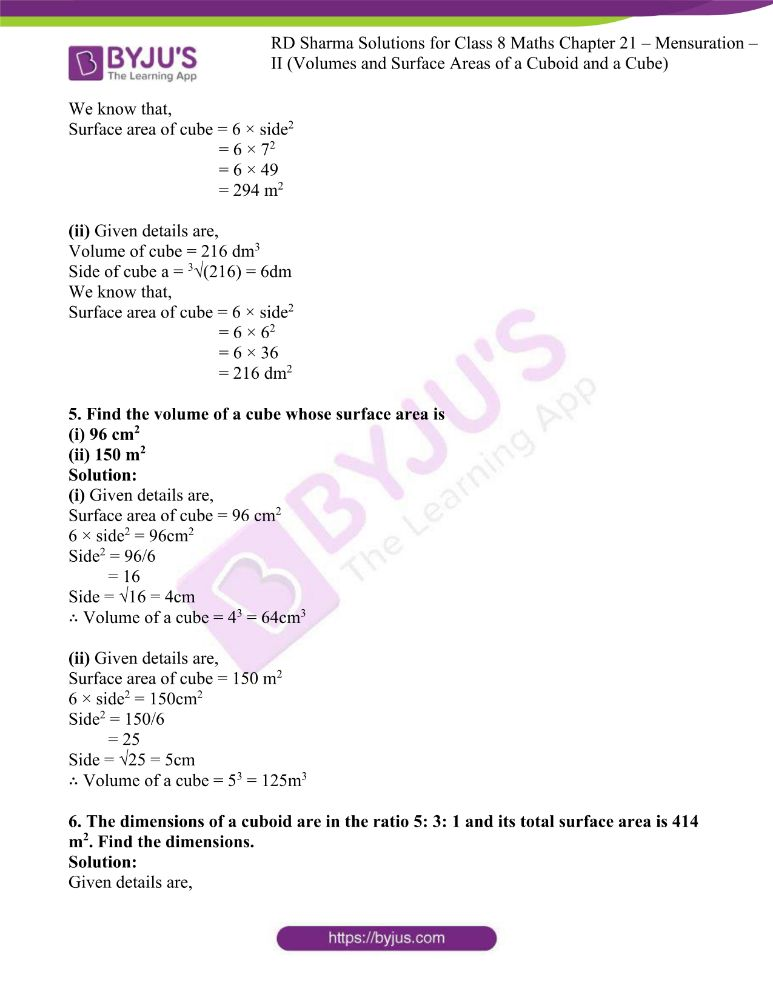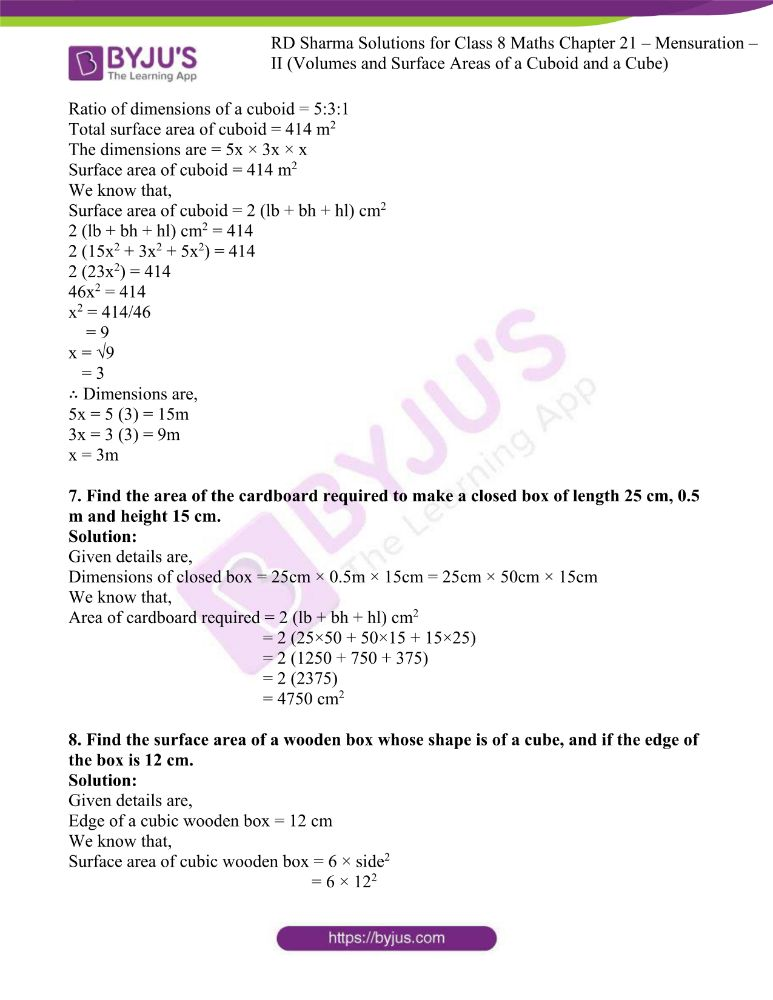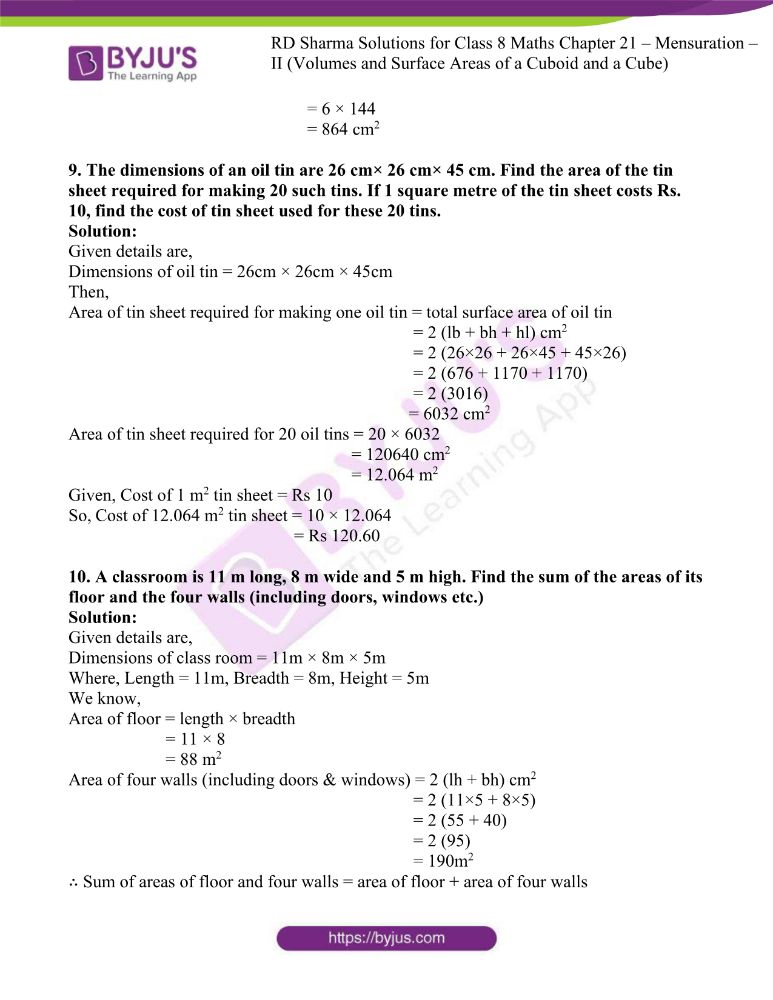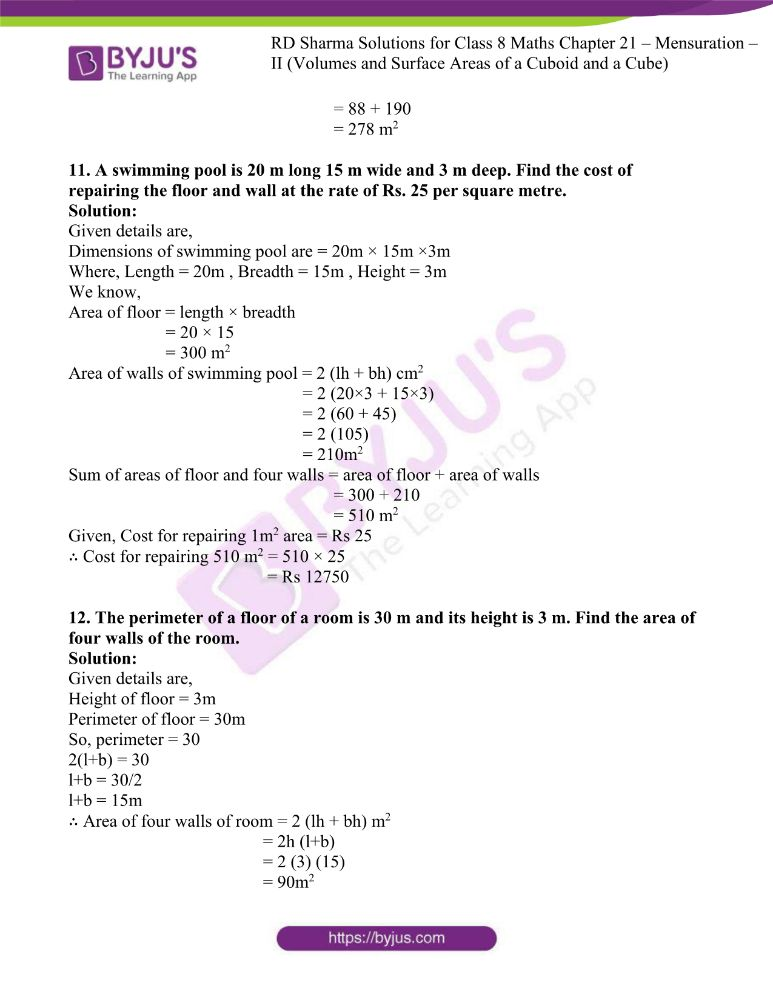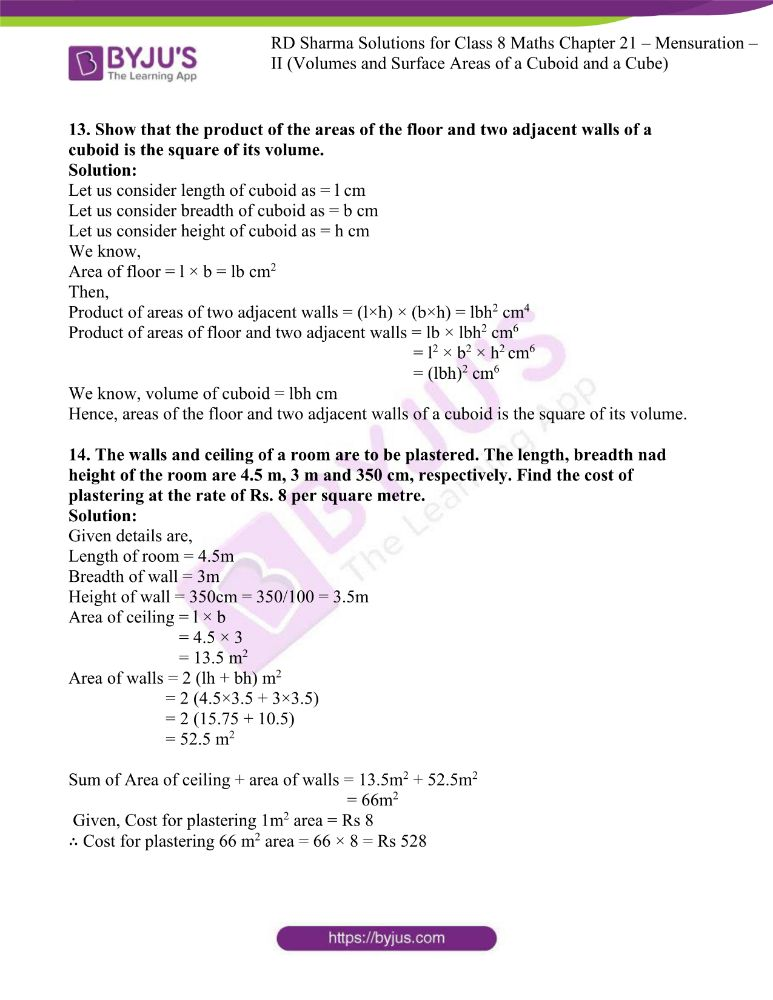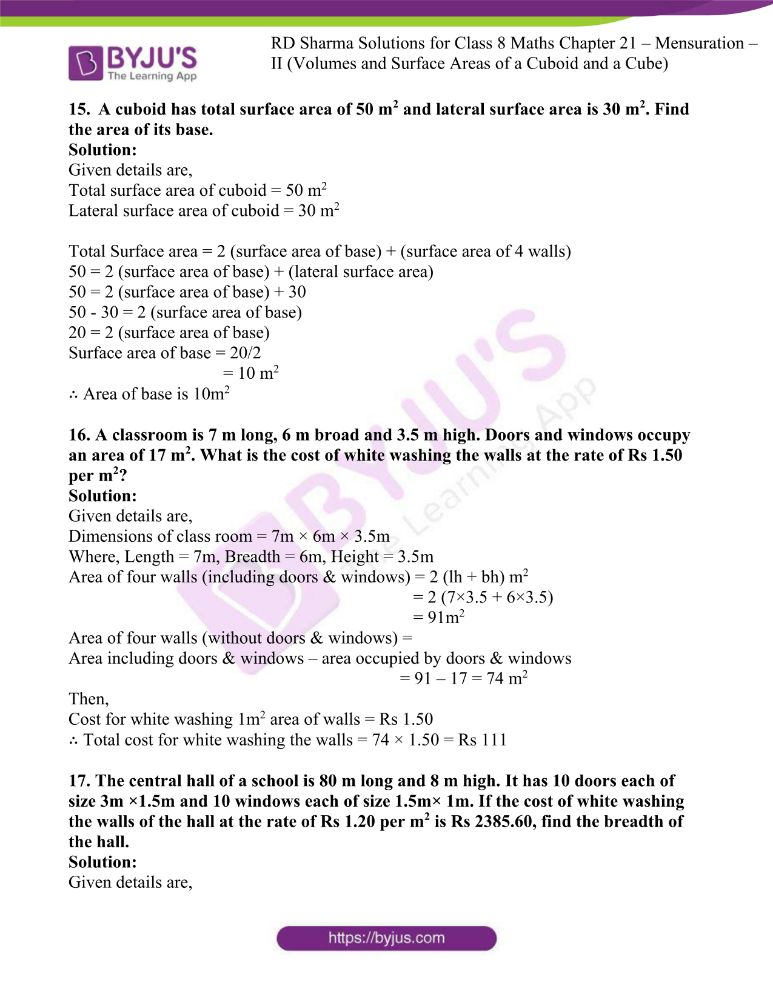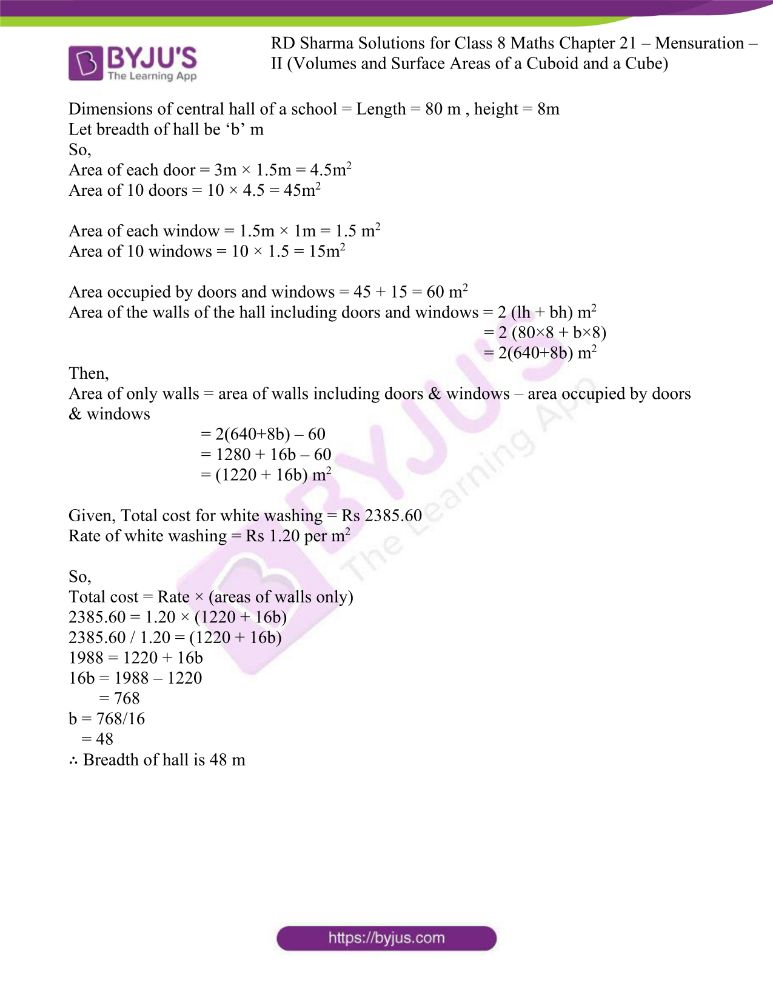### Access answers to RD Sharma Maths Solutions For Class 8 Exercise 21.3 Chapter 21 Mensuration – II (Volumes and Surface Areas of a Cuboid and a Cube)

1. Find the surface area of a cuboid whose

(i) length = 10 cm, breadth = 12 cm, height = 14 cm
(ii) length = 6 dm, breadth = 8 dm, height = 10 dm
(iii) length = 2m, breadth = 4 m, height = 5 m
(iv) length = 3.2 m, breadth = 30 dm, height = 250 cm.

Solution:

(i) Given details are,

Length of a cuboid = 10 cm

Breadth of a cuboid = 12 cm

Height of a cuboid = 14 cm

We know that,

Surface area of cuboid = 2 (lb + bh + hl) cm2

= 2 (10×12 + 12×14 + 14×10)

= 2 (120 + 168 + 140)

= 2 (428)

= 856 cm2

(ii) Given details are,

Length of a cuboid = 6 dm

Breadth of a cuboid = 8 dm

Height of a cuboid = 10 dm

We know that,

Surface area of cuboid = 2 (lb + bh + hl) cm2

= 2 (6×8 + 8×10 + 10×6)

= 2 (48 + 80 + 60)

= 2 (188)

= 376 dm2

(iii) Given details are,

Length of a cuboid = 2m

Breadth of a cuboid = 4m

Height of a cuboid = 5m

We know that,

Surface area of cuboid = 2 (lb + bh + hl) cm2

= 2 (2×4 + 4×5 + 5×2)

= 2 (8 + 20 + 10)

= 2 (38)

= 76 m2

(iv) Given details are,

Length of a cuboid = 3.2 m= 32 dm

Breadth of a cuboid = 30 dm

Height of a cuboid = 250 cm= 25 dm

We know that,

surface area of cuboid = 2 (lb + bh + hl) cm2

= 2 (32×30 + 30×25 + 25×32)

= 2 (960 + 750 + 800)

= 2 (2510)

= 5020 dm2

2. Find the surface area of a cube whose edge is
(i) 1.2 m
(ii) 27 cm
(iii) 3 cm
(iv) 6 m
(v) 2.1 m

Solution:

(i) Given,

Edge of cube = 1.2 m

We know that,

Surface area of cube = 6 × side2

= 6 × 1.22

= 6 × 1.44

= 8.64 m2

(ii) Given,

Edge of cube = 27 cm

We know that,

Surface area of cube = 6 × side2

= 6 × 272

= 6 × 729

= 4374 cm2

(iii) Given,

Edge of cube = 3 cm

We know that,

Surface area of cube = 6 × side2

= 6 × 32

= 6 × 9

= 54 cm2

(iv) Given,

Edge of cube = 6 m

We know that,

Surface area of cube = 6 × side2

= 6 × 62

= 6 × 36

= 216 m2

(v) Given,

Edge of cube = 2.1 m

We know that,

Surface area of cube = 6 × side2

= 6 × 2.12

= 6 × 4.41

= 26.46 m2

3. A cuboidal box is 5 cm by 5 cm by 4 cm. Find its surface area.

Solution:

Given details are,

Dimensions of cuboidal box = 5cm × 5cm × 4cm

We know that,

Surface area of cuboid = 2 (lb + bh + hl) cm2

= 2 (5×5 + 5×4 + 4×5)

= 2 (25 + 20 + 20)

= 2 (65)

= 130 cm2

4. Find the surface area of a cube whose volume is
(i) 343 m3
(ii) 216 dm3

Solution:

(i) Given details are,

Volume of cube = 343 m3

Side of cube, a = 3√(343) = 7m

We know that,

Surface area of cube = 6 × side2

= 6 × 72

= 6 × 49

= 294 m2

(ii) Given details are,

Volume of cube = 216 dm3

Side of cube a = 3√(216) = 6dm

We know that,

Surface area of cube = 6 × side2

= 6 × 62

= 6 × 36

= 216 dm2

5. Find the volume of a cube whose surface area is

(i) 96 cm2
(ii) 150 m2

Solution:

(i) Given details are,

Surface area of cube = 96 cm2

6 × side2 = 96cm2

Side2 = 96/6

= 16

Side = √16 = 4cm

∴ Volume of a cube = 43 = 64cm3

(ii) Given details are,

Surface area of cube = 150 m2

6 × side2 = 150cm2

Side2 = 150/6

= 25

Side = √25 = 5cm

∴ Volume of a cube = 53 = 125m3

6. The dimensions of a cuboid are in the ratio 5: 3: 1 and its total surface area is 414 m2. Find the dimensions.

Solution:

Given details are,

Ratio of dimensions of a cuboid = 5:3:1

Total surface area of cuboid = 414 m2

The dimensions are = 5x × 3x × x

Surface area of cuboid = 414 m2

We know that,

Surface area of cuboid = 2 (lb + bh + hl) cm2

2 (lb + bh + hl) cm2 = 414

2 (15x2 + 3x2 + 5x2) = 414

2 (23x2) = 414

46x2 = 414

x2 = 414/46

= 9

x = √9

= 3

∴ Dimensions are,

5x = 5 (3) = 15m

3x = 3 (3) = 9m

x = 3m

7. Find the area of the cardboard required to make a closed box of length 25 cm, 0.5 m and height 15 cm.

Solution:

Given details are,

Dimensions of closed box = 25cm × 0.5m × 15cm = 25cm × 50cm × 15cm

We know that,

Area of cardboard required = 2 (lb + bh + hl) cm2

= 2 (25×50 + 50×15 + 15×25)

= 2 (1250 + 750 + 375)

= 2 (2375)

= 4750 cm2

8. Find the surface area of a wooden box whose shape is of a cube, and if the edge of the box is 12 cm.

Solution:

Given details are,

Edge of a cubic wooden box = 12 cm

We know that,

Surface area of cubic wooden box = 6 × side2

= 6 × 122

= 6 × 144

= 864 cm2

9. The dimensions of an oil tin are 26 cm× 26 cm× 45 cm. Find the area of the tin sheet required for making 20 such tins. If 1 square metre of the tin sheet costs Rs. 10, find the cost of tin sheet used for these 20 tins.

Solution:

Given details are,

Dimensions of oil tin = 26cm × 26cm × 45cm

Then,

Area of tin sheet required for making one oil tin = total surface area of oil tin

= 2 (lb + bh + hl) cm2

= 2 (26×26 + 26×45 + 45×26)

= 2 (676 + 1170 + 1170)

= 2 (3016)

= 6032 cm2

Area of tin sheet required for 20 oil tins = 20 × 6032

= 120640 cm2

= 12.064 m2

Given, Cost of 1 m2 tin sheet = Rs 10

So, Cost of 12.064 m2 tin sheet = 10 × 12.064

= Rs 120.60

10. A classroom is 11 m long, 8 m wide and 5 m high. Find the sum of the areas of its floor and the four walls (including doors, windows etc.)

Solution:

Given details are,

Dimensions of class room = 11m × 8m × 5m

Where, Length = 11m, Breadth = 8m, Height = 5m

We know,

Area of floor = length × breadth

= 11 × 8

= 88 m2

Area of four walls (including doors & windows) = 2 (lh + bh) cm2

= 2 (11×5 + 8×5)

= 2 (55 + 40)

= 2 (95)

= 190m2

∴ Sum of areas of floor and four walls = area of floor + area of four walls

= 88 + 190

= 278 m2

11. A swimming pool is 20 m long 15 m wide and 3 m deep. Find the cost of repairing the floor and wall at the rate of Rs. 25 per square metre.

Solution:

Given details are,

Dimensions of swimming pool are = 20m × 15m ×3m

Where, Length = 20m , Breadth = 15m , Height = 3m

We know,

Area of floor = length × breadth

= 20 × 15

= 300 m2

Area of walls of swimming pool = 2 (lh + bh) cm2

= 2 (20×3 + 15×3)

= 2 (60 + 45)

= 2 (105)

= 210m2

Sum of areas of floor and four walls = area of floor + area of walls

= 300 + 210

= 510 m2

Given, Cost for repairing 1m2 area = Rs 25

∴ Cost for repairing 510 m2 = 510 × 25

= Rs 12750

12. The perimeter of a floor of a room is 30 m and its height is 3 m. Find the area of four walls of the room.

Solution:

Given details are,

Height of floor = 3m

Perimeter of floor = 30m

So, perimeter = 30

2(l+b) = 30

l+b = 30/2

l+b = 15m

∴ Area of four walls of room = 2 (lh + bh) m2

= 2h (l+b)

= 2 (3) (15)

= 90m2

13. Show that the product of the areas of the floor and two adjacent walls of a cuboid is the square of its volume.

Solution:

Let us consider length of cuboid as = l cm

Let us consider breadth of cuboid as = b cm

Let us consider height of cuboid as = h cm

We know,

Area of floor = l × b = lb cm2

Then,

Product of areas of two adjacent walls = (l×h) × (b×h) = lbh2 cm4

Product of areas of floor and two adjacent walls = lb × lbh2 cm6

= l2 × b2 × h2 cm6

= (lbh)2 cm6

We know, volume of cuboid = lbh cm

Hence, areas of the floor and two adjacent walls of a cuboid is the square of its volume.

14. The walls and ceiling of a room are to be plastered. The length, breadth nad height of the room are 4.5 m, 3 m and 350 cm, respectively. Find the cost of plastering at the rate of Rs. 8 per square metre.

Solution:

Given details are,

Length of room = 4.5m

Height of wall = 350cm = 350/100 = 3.5m

Area of ceiling = l × b

= 4.5 × 3

= 13.5 m2

Area of walls = 2 (lh + bh) m2

= 2 (4.5×3.5 + 3×3.5)

= 2 (15.75 + 10.5)

= 52.5 m2

Sum of Area of ceiling + area of walls = 13.5m2 + 52.5m2

= 66m2

Given, Cost for plastering 1m2 area = Rs 8

∴ Cost for plastering 66 m2 area = 66 × 8 = Rs 528

15. A cuboid has total surface area of 50 m2 and lateral surface area is 30 m2. Find the area of its base.

Solution:

Given details are,

Total surface area of cuboid = 50 m2

Lateral surface area of cuboid = 30 m2

Total Surface area = 2 (surface area of base) + (surface area of 4 walls)

50 = 2 (surface area of base) + (lateral surface area)

50 = 2 (surface area of base) + 30

50 – 30 = 2 (surface area of base)

20 = 2 (surface area of base)

Surface area of base = 20/2

= 10 m2

∴ Area of base is 10m2

16. A classroom is 7 m long, 6 m broad and 3.5 m high. Doors and windows occupy an area of 17 m2. What is the cost of white washing the walls at the rate of Rs 1.50 per m2?

Solution:

Given details are,

Dimensions of class room = 7m × 6m × 3.5m

Where, Length = 7m, Breadth = 6m, Height = 3.5m

Area of four walls (including doors & windows) = 2 (lh + bh) m2

= 2 (7×3.5 + 6×3.5)

= 91m2

Area of four walls (without doors & windows) =

Area including doors & windows – area occupied by doors & windows

= 91 – 17 = 74 m2

Then,

Cost for white washing 1m2 area of walls = Rs 1.50

∴ Total cost for white washing the walls = 74 × 1.50 = Rs 111

17. The central hall of a school is 80 m long and 8 m high. It has 10 doors each of size 3m ×1.5m and 10 windows each of size 1.5m× 1m. If the cost of white washing the walls of the hall at the rate of Rs 1.20 per m2 is Rs 2385.60, find the breadth of the hall.

Solution:

Given details are,

Dimensions of central hall of a school = Length = 80 m , height = 8m

Let breadth of hall be ‘b’ m

So,

Area of each door = 3m × 1.5m = 4.5m2

Area of 10 doors = 10 × 4.5 = 45m2

Area of each window = 1.5m × 1m = 1.5 m2

Area of 10 windows = 10 × 1.5 = 15m2

Area occupied by doors and windows = 45 + 15 = 60 m2

Area of the walls of the hall including doors and windows = 2 (lh + bh) m2

= 2 (80×8 + b×8)

= 2(640+8b) m2

Then,

Area of only walls = area of walls including doors & windows – area occupied by doors & windows

= 2(640+8b) – 60

= 1280 + 16b – 60

= (1220 + 16b) m2

Given, Total cost for white washing = Rs 2385.60

Rate of white washing = Rs 1.20 per m2

So,

Total cost = Rate × (areas of walls only)

2385.60 = 1.20 × (1220 + 16b)

2385.60 / 1.20 = (1220 + 16b)

1988 = 1220 + 16b

16b = 1988 – 1220

= 768

b = 768/16

= 48

∴ Breadth of hall is 48 m